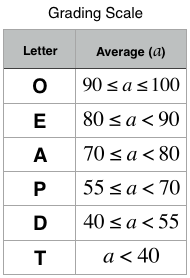Latest news:

# Hacker Rank: Day 12: InheritanceObjective

Today, we’re delving into Inheritance.

You are given two classes, Person and Student, where Person is the base class and Student is the derived class.

Completed code for Person and a declaration for Student are provided for you in the editor.

Observe that Student inherits all the properties of Person.

Complete the Student class by writing the following:

• A Student class constructor, which has 4 parameters:
1. A string, firstName.
2. A string, lastName.
3. An integer, idNumber.
4. An integer array (or vector) of test scores, scores.
• A char calculate() method that calculates a Student object’s average and returns the grade character representative of their calculated average:Input Format

The locked stub code in the editor reads the input and calls the Student class constructor with the necessary arguments.

It also calls the calculate method which takes no arguments.

The first line contains firstname, lastName, and idNumber, separated by a space.

The second line contains the number of test scores. The third line of space-separated integers describes scores.

Constraints

• 1 length of firstName, length of lastName 10
• length of idNumber = 7
• 0 score <= 100

Output Format

Output is handled by the locked stub code. Your output will be correct if your Student class constructor and calculate() method are properly implemented.

Sample Input

```Heraldo Memelli 8135627
2
100 80
```

Sample Output

```Name: Memelli, Heraldo
ID: 8135627

Explanation

This student had 2 scores to average: 100 and 80. The student’s average grade is (100 + 800) / 2 = 90.

An average grade of 90 corresponds to the letter grade O, so the calculate() method should return the character'O'.

Solution – Day 12: Inheritance

PHP Solution:

```class Student extends person {
private \$testScores;
/*
*   Class Constructor
*
*   Parameters:
*   firstName - A string denoting the Person's first name.
*   lastName - A string denoting the Person's last name.
*   id - An integer denoting the Person's ID number.
*   scores - An array of integers denoting the Person's test scores.
*/
public function __construct(\$firstName,\$lastName,\$id, \$scores){
\$this->testScores = \$scores;
\$this->id = \$id;
\$this->firstName = \$firstName;
\$this->lastName = \$lastName;
}
/*
*   Function Name: calculate
*   Return: A character denoting the grade.
*/
public function calculate(){
\$totalScore = 0;
for (\$i = 0; \$i < count(\$this->testScores); \$i++) {
\$totalScore += \$this->testScores[\$i];
}
\$avgScore = \$totalScore / count(\$this->testScores);
if (\$avgScore >= 90 && \$avgScore <= 100) {
return "O";
} else if (\$avgScore >= 80 && \$avgScore < 90) {
return "E";
} else if (\$avgScore >= 70 && \$avgScore < 80) {
return "A";
} else if (\$avgScore >= 55 && \$avgScore < 70) {
return "P";
} else if (\$avgScore >= 40 && \$avgScore < 55) {
return "D";
} else if (\$avgScore < 40) {
return "T";
}
}
}
```

Views : 167